Function Repository Resource:

# ReversibleCellularAutomaton

Generate the evolution of a reversible cellular automaton

Contributed by: Wolfram Staff
 ResourceFunction["ReversibleCellularAutomaton"][rule,init,t] generates a list representing the evolution of the reversible cellular automaton with the specified rule from initial condition init for t steps. ResourceFunction["ReversibleCellularAutomaton"][rule,init] gives the result of evolving init for one step. ResourceFunction["ReversibleCellularAutomaton"][rule] is an operator form of ResourceFunction["ReversibleCellularAutomaton"] that represents one step of evolution.

## Details

rule is taken to be an integer between 0 and 255, representing a reversible cellular automaton with 2 colors and range 1.
init is taken to be a list of two vectors of the same length with entries equal to 0 or 1.
The two initial conditions are considered to be steps 0 and 1.
ResourceFunction["ReversibleCellularAutomaton"][rule][init] is equivalent to ResourceFunction["ReversibleCellularAutomaton"][rule,init].

## Examples

### Basic Examples (2)

Run rule 30R for three steps:

 In:=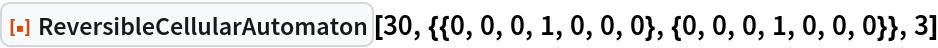Out=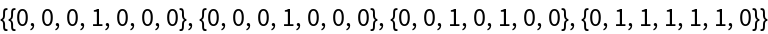Visualize the evolution of rule 30R:

 In:=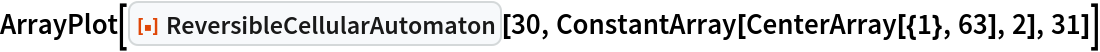Out=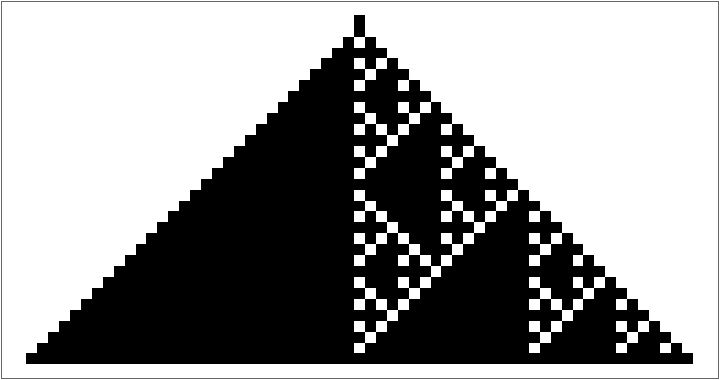### Scope (2)

Give the result of one step of rule 13R evolution:

 In:=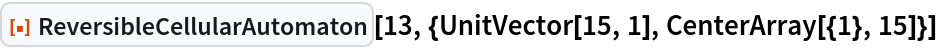Out=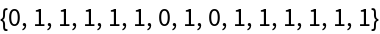An alternative form:

 In:=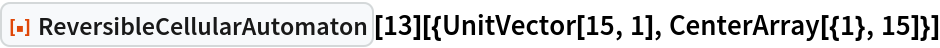Out=### Neat Examples (1)

Visualize the evolution of rule 90R with random initial conditions:

 In:=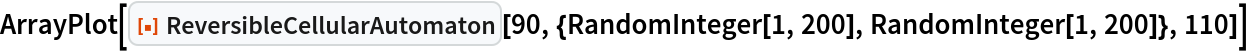Out=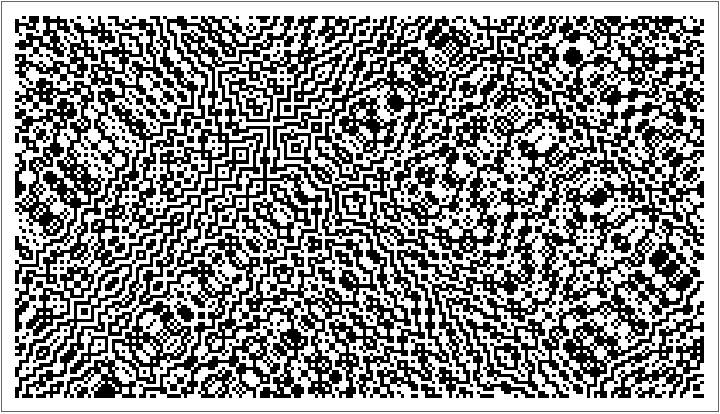## Version History

• 1.0.0 – 31 August 2021